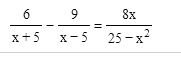User Name Remember Me? Password

 Algebra Pre-Algebra and Basic Algebra Math Forum

 November 2nd, 2014, 09:53 PM #1 Newbie   Joined: Jun 2014 From: U.S.A Posts: 13 Thanks: 0 This equation has me stumpedThis is what I basically did: 25-x^2=(x+5)(x-5) which means multiply 6 by x-5 = 6x - 30. Multiply -9 by X+5 = -9x - 45. Combine them gets me -75-3x=8x. Add 3 gets 11x/-75. giving me a final answer of -75/11 What did i do wrong?!?! Last edited by HelpMeNow; November 2nd, 2014 at 10:14 PM.November 2nd, 2014, 10:17 PM   #2
Senior Member

Joined: Jan 2012
From: Erewhon

Posts: 245
Thanks: 112

Quote:
 Originally Posted by HelpMeNowThis is what I basically did: 25-x^2=(x+5)(x-5) which means multiply 6 by x-5 = 6x - 30. Multiply -9 by X+5 = -9x - 45. Combine them gets me -75-3x=8x. Add 3 gets 11x/-75. What did i do wrong?!?!
$$\frac{6}{x+5}-\frac{9}{x-5}=\frac{6(x-5)-9(x+5)}{x^2-25}=\frac{-3x-75}{x^2-25}$$

So:
$$\frac{-3x-75}{x^2-25}=\frac{8x}{25-x^2}=\frac{-8x}{x^2-25}$$

So if $x^2-25 \ne 0$, you have $-3x-75=-8x$

CBNovember 2nd, 2014, 10:45 PM   #3
Newbie

Joined: Jun 2014
From: U.S.A

Posts: 13
Thanks: 0

Quote:
 Originally Posted by CaptainBlack$$\frac{6}{x+5}-\frac{9}{x-5}=\frac{6(x-5)-9(x+5)}{x^2-25}=\frac{-3x-75}{x^2-25}$$ So: $$\frac{-3x-75}{x^2-25}=\frac{8x}{25-x^2}=\frac{-8x}{x^2-25}$$ So if $x^2-25 \ne 0$, you have $-3x-75=-8x$ CB
What?November 2nd, 2014, 11:13 PM   #4
Senior Member

Joined: Apr 2013

Posts: 425
Thanks: 24

Quote:
 Originally Posted by HelpMeNowWhat?
What "What"????November 3rd, 2014, 01:38 AM   #5
Senior Member

Joined: Jan 2012
From: Erewhon

Posts: 245
Thanks: 112

Quote:
 Originally Posted by HelpMeNowWhat?
Your explanation of what you did is incomprehensible, as far as I can tell you lost a minus sign in the wash and you did not observe that you had to assume that $x^2\ne 5$ as otherwise both sides of the equation you are trying to solve are undefined.

CBNovember 3rd, 2014, 02:35 AM   #6
Math Team

Joined: Apr 2010

Posts: 2,780
Thanks: 361

Quote:
 Originally Posted by CaptainBlack you had to assume that $\displaystyle x^2\ne 5$
(Presumably, you meant $\displaystyle x^2\ne 25$)November 3rd, 2014, 04:56 AM   #7
Senior Member

Joined: Jan 2012
From: Erewhon

Posts: 245
Thanks: 112

Quote:
 Originally Posted by Hoempa(Presumably, you meant $\displaystyle x^2\ne 25$)
Yes, it was/is correct in the original response.

CBNovember 3rd, 2014, 05:00 AM   #8
Senior Member

Joined: Apr 2014
From: Glasgow

Posts: 2,157
Thanks: 732

Math Focus: Physics, mathematical modelling, numerical and computational solutions
Quote:
 Originally Posted by HelpMeNowWhat?
$\displaystyle \frac{8x}{25 - x^2} = \frac{8x}{-(x^2 - 25)} = \frac{-8x}{x^2 - 25}$

This is so that the denominators on the LHS and RHS are exactly equivalent, so you can equate the numerators. Consequently, you have $\displaystyle -3x - 75 = -8x$ instead of $\displaystyle -3x-75 = 8x$.

Last edited by Benit13; November 3rd, 2014 at 05:02 AM.Tags equation, stumpedThread ToolsShow Printable VersionEmail this Page Display ModesLinear ModeSwitch to Hybrid ModeSwitch to Threaded ModeSimilar Threads Thread Thread Starter Forum Replies Last Post Vitals Algebra 1 August 29th, 2014 10:24 AM mmcdonald Calculus 3 March 1st, 2012 05:28 AM tangosix Algebra 2 March 2nd, 2010 10:39 AM yabo2k Advanced Statistics 1 October 27th, 2009 05:41 AM bretttolbert Algebra 4 April 5th, 2008 12:15 PM

 Contact - Home - Forums - Cryptocurrency Forum - Top

Copyright © 2019 My Math Forum. All rights reserved.